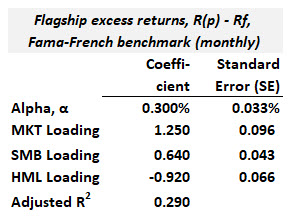# P2.T9.21.1. Factor regression and style analysis

#### David Harper CFA FRM

##### David Harper CFA FRM
Staff member
Subscriber
Learning outcomes: Describe Grinold’s fundamental law of active management, including its assumptions and limitations, and calculate the information ratio using this law. Apply a factor regression to construct a benchmark with multiple factors, measure a portfolio’s sensitivity to those factors, and measure alpha against that benchmark. Explain how to use style analysis to handle time-varying factor exposures.

Questions:

21.1.1. Patty the portfolio manager has an information coefficient (IC) equal to 0.150 and a breadth (BR) equal to 12.0. If she triples her breadth to 36, what happens to the information ratio (IR)?

a. The IR drops by 1/3rd to 0.346
b. It has no effect on the IR
c. The IR increases by 0.380 to 0.900
d. The IR increases by 1.039 to 1.559

21.1.2. Richard conducted a factor regression for his firm's Flagship Portfolio. He used the three-factor Fama-French specification where the factors are MKT (the market), SMB (small stocks minus big stocks), and HML (value stocks minus growth stocks). His factor regression results are displayed below.Please note the regression is based on monthly returns. Each of the following statements is TRUE about these factor regression results EXCEPT which is false?

a. The portfolio's style appears to growth-oriented, small-cap
a. This portfolio manager(s) appear to be skillful; that is, the alpha is significant
c. The low adjusted R^2 and low standard errors imply these factors are not appropriate to this portfolio
d. The implied CAPM benchmark is leveraged as it borrows cash (i.e., T-bills) to invest more than 100% (i.e., > \$1.0) in the market portfolio

21.1.3. In contrast to factor regressions that assume constant factor weights, which of the following is the primary advantage of style analysis to determine the factor benchmark?

a. The factor benchmark uses actual tradeable funds
b. The factor benchmark can include a momentum factor
c. The factor benchmark naturally overcomes the beta anomaly
d. Statistical inference is easier when the factor loadings vary over time